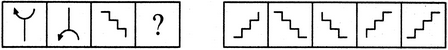# Non Verbal Reasoning - Analogy - Discussion

### Discussion :: Analogy - Section 1 (Q.No.3)

Each of the following questions consists of two sets of figures. Figures A, B, C and D constitute the Problem Set while figures 1, 2, 3, 4 and 5 constitute the Answer Set. There is a definite relationship between figures A and B. Establish a similar relationship between figures C and D by selecting a suitable figure from the Answer Set that would replace the question mark (?) in fig. (D).

3.

Select a suitable figure from the Answer Figures that would replace the question mark (?).(A)     (B)      (C)     (D)                  (1)      (2)      (3)      (4)      (5)

 [A]. 1 [B]. 2 [C]. 3 [D]. 4 [E]. 5

Explanation:

The figure gets vertically inverted.

 Thaya (Muthiah) said: (Oct 6, 2013) First figure 180 degree shifted in second figure if consider third one. It must be 180 degree shift in answer.

 Karthik R said: (Aug 12, 2014) Option D is the right one. If it is shifted to 180 degree to the left then option D will be the closer answer. To that.

 Vilas Kumar said: (Nov 11, 2015) Please give me explanation.

 Jeeva said: (Apr 9, 2016) I can't understand this question. Please explain me to understand quickly.

 Harshit said: (Oct 5, 2016) C is right as it should shift 180 degrees.

 Hassan Ak said: (Nov 9, 2016) It is rotated 180 degree.

 Phil said: (Nov 11, 2016) No, it flips upside down.

 Tejas said: (Jan 20, 2017) I feel C is the right answer as it would get inverted as in A to B.

 Ritu said: (Feb 8, 2017) In different ways we can give the answer of this question. But I feel A is the right with concern to water image.

 Clock Sid said: (Apr 5, 2017) First, take mirror image then flip 180 degree that is pattern flipping 180 degree we get c option then flipping 180 degree we get an option it is simple.

 Kruti said: (Jul 15, 2017) Consider it as stairs. Figure A and Figure C is taking you Upside and Figure B suggests going downside. Now if Figure c has first vertical line (bottom to top approach- upside) then in figure D the first line should also be vertical (top to bottom approach - downside). Hence Option A is the correct one.

 Anurag said: (Feb 15, 2018) C is the right answer.

 Ishant said: (Jan 29, 2020) C is the correct answer. I too agree.

 Ishan said: (Jan 29, 2020) Option A is right because when we turn top to bottom.

 Chandra said: (Feb 14, 2020) C is the right answer.

 Aken said: (Dec 12, 2020) If this is water image then A option is correct otherwise C is correct.

 Gyanu said: (Jul 17, 2021) Option D is the correct answer 180 degree rotation.

 Vicky said: (Aug 3, 2021) A is correct. It is vertically inverted (flipped upside down).